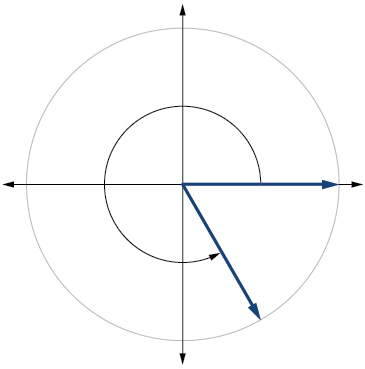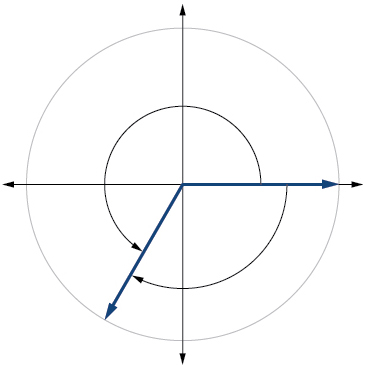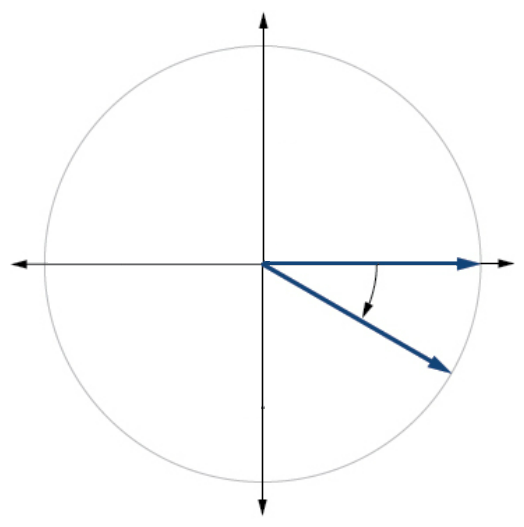$$\newcommand{\id}{\mathrm{id}}$$ $$\newcommand{\Span}{\mathrm{span}}$$ $$\newcommand{\kernel}{\mathrm{null}\,}$$ $$\newcommand{\range}{\mathrm{range}\,}$$ $$\newcommand{\RealPart}{\mathrm{Re}}$$ $$\newcommand{\ImaginaryPart}{\mathrm{Im}}$$ $$\newcommand{\Argument}{\mathrm{Arg}}$$ $$\newcommand{\norm}{\| #1 \|}$$ $$\newcommand{\inner}{\langle #1, #2 \rangle}$$ $$\newcommand{\Span}{\mathrm{span}}$$

# 5.1e: Exercises - Angles

$$\newcommand{\vecs}{\overset { \rightharpoonup} {\mathbf{#1}} }$$ $$\newcommand{\vecd}{\overset{-\!-\!\rightharpoonup}{\vphantom{a}\smash {#1}}}$$$$\newcommand{\id}{\mathrm{id}}$$ $$\newcommand{\Span}{\mathrm{span}}$$ $$\newcommand{\kernel}{\mathrm{null}\,}$$ $$\newcommand{\range}{\mathrm{range}\,}$$ $$\newcommand{\RealPart}{\mathrm{Re}}$$ $$\newcommand{\ImaginaryPart}{\mathrm{Im}}$$ $$\newcommand{\Argument}{\mathrm{Arg}}$$ $$\newcommand{\norm}{\| #1 \|}$$ $$\newcommand{\inner}{\langle #1, #2 \rangle}$$ $$\newcommand{\Span}{\mathrm{span}}$$ $$\newcommand{\id}{\mathrm{id}}$$ $$\newcommand{\Span}{\mathrm{span}}$$ $$\newcommand{\kernel}{\mathrm{null}\,}$$ $$\newcommand{\range}{\mathrm{range}\,}$$ $$\newcommand{\RealPart}{\mathrm{Re}}$$ $$\newcommand{\ImaginaryPart}{\mathrm{Im}}$$ $$\newcommand{\Argument}{\mathrm{Arg}}$$ $$\newcommand{\norm}{\| #1 \|}$$ $$\newcommand{\inner}{\langle #1, #2 \rangle}$$ $$\newcommand{\Span}{\mathrm{span}}$$

### A: Concepts

Exercise $$\PageIndex{A}$$

1) Draw an angle in standard position. Label the vertex, initial side, and terminal side.

2) Explain why there are an infinite number of angles that are coterminal to a certain angle.

3) State what a positive or negative angle signifies, and explain how to draw each.

4) How does radian measure of an angle compare to the degree measure? Include an explanation of $$1$$ radian in your paragraph.

1.3. Whether the angle is positive or negative determines the direction. A positive angle is drawn in the counterclockwise direction, and a negative angle is drawn in the clockwise direction.

### B: Draw Angles in Standard Position

Exercise $$\PageIndex{B}$$

$$\bigstar$$ Draw an angle in standard position with the given measure. If the angle measure is not between $$0$$ and $$2 \pi$$ or between $$0^{\circ}$$ and $$360^{\circ}$$, also state the coterminal angle that is within that interval.

 5. $$300^{\circ}$$ 6. $$415^{\circ}$$ 7. $$135^{\circ}$$ 8. $$-30^{\circ}$$ 9. $$-120^{\circ}$$ 10. $$-315^{\circ}$$ 11. $$\dfrac{2π}{3}$$ 12.  $$\dfrac{5π}{3}$$ 13. $$−\dfrac{4π}{3}$$ 14. $$−\dfrac{5π}{3}$$ 15. $$\dfrac{5π}{6}$$ 16.  $$\dfrac{11π}{6}$$ 17. $$−\dfrac{π}{6}$$ 18. $$\dfrac{7π}{6}$$ 19. $$\dfrac{7π}{4}$$ 20. $$\dfrac{5π}{4}$$ 21. $$−\dfrac{3π}{4}$$ 22. $$\dfrac{11π}{4}$$ 23. $$\dfrac{22π}{3}$$ 24. $$\dfrac{17π}{6}$$ 25. $$−\dfrac{π}{10}$$ 26. $$\dfrac{23π}{5}$$ 27. $$\dfrac{π}{2}$$ 28. $$-\dfrac{3π}{2}$$ 29. $$\dfrac{7π}{2}$$ 30. $$-5π$$
 5.7.9.   $$240^{\circ}$$11.13. $$\frac{2π}{3}$$x 15.17.19.21.23. $$\frac{4π}{3}$$25. $$\frac{19π}{10}$$27.29 .  $$\frac{3π}{2}$$### C: Convert between radians and degrees

Exercise $$\PageIndex{C}$$

$$\bigstar$$ Convert angles in radians to degrees.

 36. $$\dfrac{3π}{4}$$ radians   37. $$\dfrac{π}{9}$$ radians 38. $$−\dfrac{5π}{4}$$ radians   39. $$\dfrac{π}{3}$$ radians 40. $$−\dfrac{7π}{3}$$ radians   41. $$−\dfrac{5π}{12}$$ radians 42. $$\dfrac{11π}{6}$$ radians

$$\bigstar$$ Convert angles in degrees to radians. Write the answer both as a multiple of pi and to the nearest hundredth of a radian.

 43. $$90^{\circ}$$ 44. $$100^{\circ}$$ 45. $$-540^{\circ}$$ 46. $$-120^{\circ}$$ 47. $$180^{\circ}$$ 48. $$-315^{\circ}$$ 49. $$150^{\circ}$$

37. $$20^{\circ} \qquad$$ 39. $$60^{\circ} \qquad$$ 41. $$-75^{\circ} \qquad$$ 43. $$\frac{π}{2} \; \approx \; 1.57$$ radians  $$\qquad$$ 45. $$−3π \; \approx \; 9.42$$ radians
47. $$π \; \approx \; 3.14$$ radians $$\qquad$$ 49. $$\frac{5π}{6} \; \approx \; 2.62$$ radians

### D: Coterminal Angles

Exercise $$\PageIndex{D}$$

$$\bigstar$$ Find the angle between $$0^{\circ}$$ and $$360^{\circ}$$ that is coterminal to the given angle.

50. $$-40^{\circ}$$     $$\qquad$$    51. $$-110^{\circ}$$     $$\qquad$$    52. $$700^{\circ}$$     $$\qquad$$    53. $$1400^{\circ}$$

$$\bigstar$$ Find the angle between $$0$$ and $$2\pi$$ in radians that is coterminal to the given angle.

54. $$−\dfrac{π}{9}$$     $$\qquad$$    55. $$\dfrac{10π}{3}$$     $$\qquad$$    56. $$\dfrac{13π}{6}$$     $$\qquad$$    57. $$\dfrac{44π}{9}$$

51. $$250^{\circ}$$     $$\qquad$$    53. $$320^{\circ}$$     $$\qquad$$ 55. $$\frac{4π}{3}$$     $$\qquad$$    57. $$\frac{8π}{9}$$